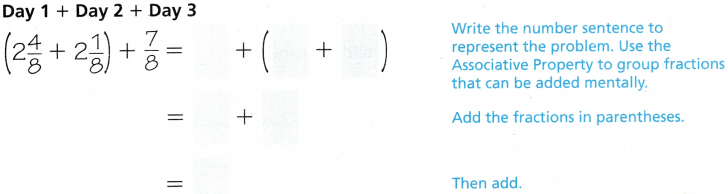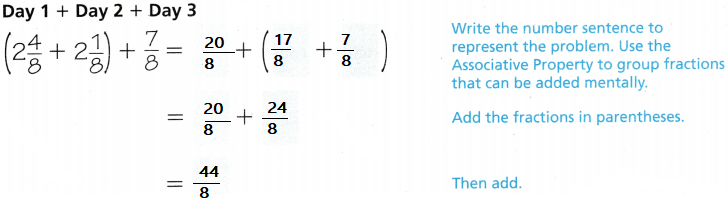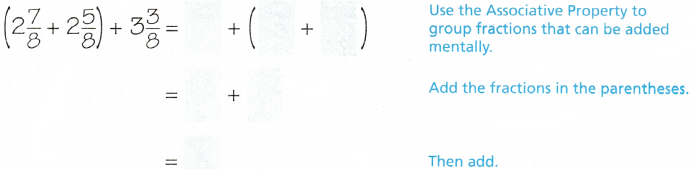Refer to our Texas Go Math Grade 4 Answer Key Pdf to score good marks in the exams. Test yourself by practicing the problems from Texas Go Math Grade 4 Lesson 5.7 Answer Key Use Properties of Addition.

Essential Question

the commutative property of addition states that when finding a sum, changing the order of the addends will not change their sum. In symbols, the commutative property of addition says that for numbers
The associative property of addition states that when finding a sum, changing the way addends are grouped will not change their sum. In symbols, the associative property of addition says that for numbers

Unlock the Problem

Jane and her teammates are training for their track meet. On the first day of practice, they run 2$$\frac{4}{8}$$ miles around the track. On the second day, they run 2$$\frac{1}{8}$$ miles. On the last day of practice before the meet, they run $$\frac{7}{8}$$ mile. How many miles did Jane and her teammates run to train for their track meet?

Use the Associative Property.So, Jane and her teammates ran __________ miles to prepare for their track meet.Explanation:
So, Jane and her teammates ran $$\frac{44}{8}$$ miles to prepare for their track meet.

Math Talk

Mathematical Processes
Explain why grouping the fractions differently makes it to find the sum.
Because mixed fractions are again converted to fractions
to find the sum

Explain why you would not group 2$$\frac{4}{8}$$ and $$\frac{7}{8}$$ together in parentheses.
One is fraction and the other is mixed fraction

Example Add. (2$$\frac{7}{8}$$ + 2$$\frac{5}{8}$$) + 3$$\frac{3}{8}$$

Use the Associative PropertyExplanation:
By using the associative property solved the addition sum of mixed fraction.

Try This!

Subtraction is not commutative or associative. When you subtract, perform operations in parentheses first. Then subtract from left to right.
a. 5$$\frac{8}{12}$$ – $$\frac{2}{12}$$ – $$\frac{1}{12}$$ = _______ – $$\frac{1}{12}$$ = ____________
5$$\frac{8}{12}$$ – $$\frac{2}{12}$$ – $$\frac{1}{12}$$ = 5$$\frac{6}{12}$$ – $$\frac{1}{12}$$ = 5$$\frac{5}{12}$$
Explanation:
First subtracted the whole then subtracted the fractions

b. $$\left(5 \frac{8}{12}-\frac{2}{12}\right)-\frac{1}{12}$$ = ____________ – $$\frac{1}{12}$$ = ____________
(5$$\frac{8}{12}$$ – $$\frac{2}{12}$$ )- $$\frac{1}{12}$$ =(5$$\frac{6}{12}$$)

(5$$\frac{6}{12}$$) – $$\frac{1}{12}$$ = 5$$\frac{5}{12}$$
Explanation:
First subtracted the whole then subtracted the fractions

c. $$5 \frac{8}{12}-\left(\frac{2}{12}-\frac{1}{12}\right)$$ = 5$$\frac{8}{12}$$ – ____________ = ____________
5$$\frac{8}{12}$$ – ($$\frac{2}{12}$$ – $$\frac{1}{12}$$ )= 5$$\frac{1}{12}$$
5$$\frac{8}{12}$$– $$\frac{1}{12}$$ = 5$$\frac{7}{12}$$

Explain how you can use your answers to parts b and c to conclude that subtraction is not associative.
The answer are different from part b to part c.

Share and Show

Use the properties and mental math to solve. Write your answer in simplest form.

$$\left(2 \frac{5}{8}+\frac{1}{8}\right)+\frac{7}{8}$$
$$\frac{21}{8}$$ + 1
= $$\frac{29}{8}$$
Explanation:
Used mental math’s to solve the equation into simplest form

Question 2.
$$\frac{7}{12}+\left(\frac{5}{12}+\frac{2}{12}\right)$$
$$\frac{14}{12}$$ = 2$$\frac{2}{12}$$
Explanation:
Used mental math’s to solve the equation into simplest form

Question 3.
$$3 \frac{1}{4}+\left(2 \frac{3}{4}+6 \frac{2}{4}\right)$$
$$\frac{18}{4}$$  = 4$$\frac{2}{4}$$
Explanation:
Used mental math’s to solve the equation into simplest form

Problem Solving

Use the map to solve 4-5.Question 4.
Multi-Step In the morning, Julie rides her hike from the sports complex to the school. In the afternoon, she rides from the school to the mall, and then to Kyle’s house. How far does Julie ride her bike?
$$\frac{2}{5}$$ + $$\frac{2}{5}$$ +$$\frac{4}{5}$$
$$\frac{8}{5}$$ mile far that Julie ride her bike.

Question 5.
H.O.T. On one afternoon, Mario walks from his house to the library. That evening, Mario walks from the library to the mall, and then to Kyle’s house. Describe how you can use the properties to find how far Mario walks.
Answer: By using the commutative property
Explanation:
(1$$\frac{4}{5}$$ + 1$$\frac{3}{5}$$ )+ $$\frac{4}{5}$$
2$$\frac{11}{5}$$ = 4$$\frac{1}{5}$$
4$$\frac{1}{5}$$  mile  far Mario walks.

Question 6.
H.O.T. Analyze Explain how you would use the Associative Property to help you solve this problem. $$\left(6 \frac{2}{8}+5 \frac{3}{8}\right)+1 \frac{5}{8}$$
6$$\frac{2}{8}$$+(5$$\frac{3}{8}$$+1$$\frac{5}{8}$$)
Add the fractions $$\frac{2}{8}$$+$$\frac{3}{8}$$+$$\frac{5}{8}$$
=$$\frac{10}{8}$$
=1$$\frac{2}{8}$$ = 13$$\frac{2}{8}$$

A bicycle rider trains on Type 3 hills for $$\frac{3}{8}$$ of his practice time, Type 2 hills for $$\frac{1}{8}$$ of the time, and Type HC hills for $$\frac{3}{8}$$ of the time. The rest of the practice time is spent on flat ground. What part of his practice time does the rider train on hills?
$$\frac{3}{8}$$+$$\frac{1}{8}$$+$$\frac{3}{8}$$
=$$\frac{7}{8}$$part of his practice time that the rider train on hills
Explanation:

Question 8.
Marie is making a costume. She uses $$\frac{2}{4}$$ yard of blue cloth, $$\frac{1}{4}$$ yard of green cloth, and yard of red cloth. Which shows a way Marie could find the amount of cloth she uses?
(A) $$\frac{2}{4}+\left(\frac{1}{4}+\frac{1}{4}\right)$$
(B) $$\frac{2}{4}+\left(\frac{2}{4}+\frac{1}{4}\right)$$
(C) $$\frac{3}{4}+\left(\frac{1}{4}+\frac{1}{4}\right)$$
(D) $$\frac{1}{4}+\left(\frac{1}{4}+\frac{1}{4}\right)$$
Explanation:
$$\frac{2}{4}+\left(\frac{1}{4}+\frac{1}{4}\right)$$ shows a way Marie that the amount of cloth she uses.

Question 9.
Multi-Step Otis needs 1 pound of apples to make an apple pie. He has $$\frac{3}{12}$$ pound of yellow apples, $$\frac{4}{12}$$ pound of red apples, and $$\frac{3}{12}$$ pound of green apples. How many more pounds of apples does he need?
(A) $$\frac{6}{12}$$ pound
(B) $$\frac{7}{12}$$ pound
(C) $$\frac{10}{12}$$ pound
(D) $$\frac{2}{12}$$ pound
Explanation:
$$\frac{3}{12}$$+$$\frac{4}{12}$$+$$\frac{3}{12}$$ = $$\frac{10}{12}$$
1- $$\frac{10}{12}$$ = $$\frac{2}{12}$$ pound of more apples he need

TEXAS Test Prep

Question 10.
Bill got an answer of $$\frac{8}{10}$$ to the problem $$\left(\frac{9}{10}-\frac{4}{10}\right)-\frac{3}{10}$$ Which statement shows the error he made?
(A) He did not subtract all the numbers.
(B) He subtracted $$\left(\frac{9}{10}-\frac{4}{10}\right)$$ first.
(C) He regrouped as $$\frac{9}{10}-\left(\frac{4}{10}-\frac{3}{10}\right)$$
(D) He subtracted from left to right.
Explanation:
He regrouped as $$\frac{9}{10}-\left(\frac{4}{10}-\frac{3}{10}\right)$$ this the error he made

### Texas Go Math Grade 4 Lesson 5.7 Homework and Practice Answer Key

Use the properties and mental math to solve.

Question 1.
$$\frac{1}{5}+\left(\frac{4}{5}+\frac{2}{5}\right)$$
$$\frac{1}{5}$$ + $$\frac{6}{5}$$
= $$\frac{7}{5}$$
Explanation:
Used mental math’s to solve the equation into simplest form by using the properties

Question 2.
$$\left(\frac{3}{9}+\frac{5}{9}\right)+\frac{4}{9}$$
$$\frac{8}{9}$$ + $$\frac{4}{9}$$
= $$\frac{12}{9}$$

=1$$\frac{3}{9}$$
Explanation:
Used mental math’s to solve the equation into simplest form by using the properties

Question 3.
$$\left(\frac{2}{3}+\frac{2}{3}\right)+\frac{1}{3}$$
$$\frac{4}{3}$$ + $$\frac{1}{3}$$
= $$\frac{5}{3}$$
=1$$\frac{5}{3}$$
Explanation:
Used mental math’s to solve the equation into simplest form by using the properties

$$4 \frac{2}{6}+\left(2 \frac{4}{6}+3 \frac{3}{6}\right)$$
4$$\frac{2}{6}$$ +5 $$\frac{7}{6}$$
= 9$$\frac{9}{6}$$ =10$$\frac{3}{6}$$
Explanation:
Used mental math’s to solve the equation into simplest form by using the properties

Question 5.
$$\left(2 \frac{3}{4}+1 \frac{3}{4}\right)+3 \frac{1}{4}$$
3$$\frac{6}{4}$$ + 3$$\frac{1}{4}$$
=6 $$\frac{7}{4}$$
=7$$\frac{3}{4}$$
Explanation:
Used mental math’s to solve the equation into simplest form by using the properties

Question 6.
$$1 \frac{2}{7}+\left(3 \frac{5}{7}+3 \frac{4}{7}\right)$$
1$$\frac{2}{7}$$ + 6$$\frac{9}{7}$$
= 7$$\frac{11}{7}$$

=8$$\frac{4}{7}$$
Explanation:
Used mental math’s to solve the equation into simplest form by using the properties

Problem Solving

Use the map for 7-8.Question 7.
On Saturday, Mario walked from his house to the library. Later that day, he walked from the library to the mall, and then to the park. How far did Mario walk on Saturday?
1$$\frac{4}{5}$$+1$$\frac{3}{5}$$+$$\frac{2}{5}$$
Explanation:
Added the fractions $$\frac{4+3+2}{5}$$
$$\frac{8}{5}$$ = 3$$\frac{3}{5}$$

Practice and Homework Lesson 5.7 Answer Key Question 8.
Kyle rode his bike from the library to the mall. Later he rode from the mall to school, and then to the sports complex. How far did Kyle ride his bike?
1$$\frac{3}{5}$$+$$\frac{2}{5}$$+$$\frac{2}{5}$$
Explanation:
Added the fractions $$\frac{2+3+2}{5}$$
$$\frac{7}{5}$$ = 2$$\frac{2}{5}$$

Lesson Check

Question 9.
Robin is studying for a history test. She studies for $$\frac{3}{4}$$ hour on both Friday and Saturday and 1$$\frac{1}{4}$$ hour on Sunday. Robin writes $$\left(\frac{3}{4}+\frac{3}{4}\right)+1 \frac{1}{4}$$ to find the amount of time she studied. Which shows another way Robin could find the amount of time she studied?
(A) $$\frac{3}{4}+\left(\frac{3}{4}+\frac{3}{4}\right)$$
(B) $$\frac{3}{4}+\left(\frac{3}{4}+\frac{1}{4}\right)$$
(C) $$\frac{3}{4}+\left(1 \frac{1}{4}+1 \frac{1}{4}\right)$$
(D) $$\frac{3}{4}+\left(\frac{3}{4}+1 \frac{1}{4}\right)$$
Explanation:
$$\frac{3}{4}+\left(\frac{3}{4}+1 \frac{1}{4}\right)$$is the another way that Robin could find the amount of time she studied.

Question 10.
At Hill School, the fourth grade classes each had a pizza party. Mr Dean’s class ate 5$$\frac{3}{8}$$ Mrs. Sander’s class ate 4$$\frac{5}{8}$$ pizzas, and Mrs. Carter’s class ate 5$$\frac{5}{8}$$ pizzas. What is the total amount of pizza eaten by all three classes?
(A) 14$$\frac{7}{8}$$ pizzas
(B) 14$$\frac{1}{2}$$ pizzas
(C) 15$$\frac{5}{8}$$ pizzas
(D) 15 pizzas
Explanation:
5$$\frac{3}{8}$$ + 4$$\frac{5}{8}$$ + 5$$\frac{5}{8}$$
Add the whole 5 +4+5= 14
Add the fractions $$\frac{13}{8}$$
15$$\frac{5}{8}$$

Question 11.
The distance from Jill’s house to the grocery store is 3$$\frac{7}{10}$$ miles. The distance from the grocery store to the hank is $$\frac{2}{10}$$ mile. The distance from the bank to the gym is 5$$\frac{1}{10}$$ miles. If Jill drives from her house to the bank and then to the gym, how far has she traveled?
(A) 9 miles
(B) 9$$\frac{1}{5}$$ miles
(C) 8 miles
(D) 8$$\frac{1}{5}$$ miles
Explanation:
3$$\frac{7}{10}$$+$$\frac{2}{10}$$+5$$\frac{1}{10}$$
Add the fractions $$\frac{7+2+1}{10}$$ = 1
8+1=9

At lunchtime, Dale’s Diner served a total of 2$$\frac{2}{6}$$ pots of vegetable soup, 3$$\frac{5}{6}$$ pots of chicken soup, and 4$$\frac{3}{6}$$ pots of tomato soup. I low many pots of soup were served in all?
(A) 9$$\frac{2}{3}$$ pots
(B) 10$$\frac{2}{3}$$ pots
(C) 10$$\frac{1}{3}$$ pots
(D) 9$$\frac{1}{3}$$ pots
Explanation:
2$$\frac{2}{6}$$ + 3$$\frac{5}{6}$$+4$$\frac{3}{6}$$
Add the fractions $$\frac{2+5+3}{6}$$ = $$\frac{10}{6}$$
= 10$$\frac{4}{6}$$

Question 13.
Multi-Step William wants to run a mile each afternoon to train for a race. If William runs $$\frac{3}{10}$$ of a mile, then $$\frac{4}{10}$$ of a mile and then $$\frac{2}{10}$$ of a mile, how many more miles does he need to run to reach his goal?
(A) $$\frac{6}{10}$$ mile
(B) $$\frac{9}{10}$$ mile
(C) $$\frac{1}{10}$$ mile
(D) $$\frac{7}{10}$$ mile
Explanation:
$$\frac{3}{10}$$ + $$\frac{4}{10}$$ + $$\frac{2}{10}$$
Add the fractions directly $$\frac{3+4+2}{10}$$
1-$$\frac{9}{10}$$ = $$\frac{1}{10}$$

Question 14.
Grace has 2$$\frac{1}{3}$$ yards of red fabric, 2$$\frac{2}{3}$$ yards of blue fabric, and 1$$\frac{2}{3}$$ yards of green fabric. How much fabric does Grace have?
(A) 6$$\frac{2}{3}$$ yards
(B) 5$$\frac{2}{3}$$ yards
(C) 7$$\frac{2}{3}$$ yards
(D) 3$$\frac{1}{3}$$ yards
2$$\frac{1}{3}$$ + 2$$\frac{2}{3}$$ + 1$$\frac{2}{3}$$
Add the fractions $$\frac{1+2+2}{3}$$
6$$\frac{2}{3}$$ yards